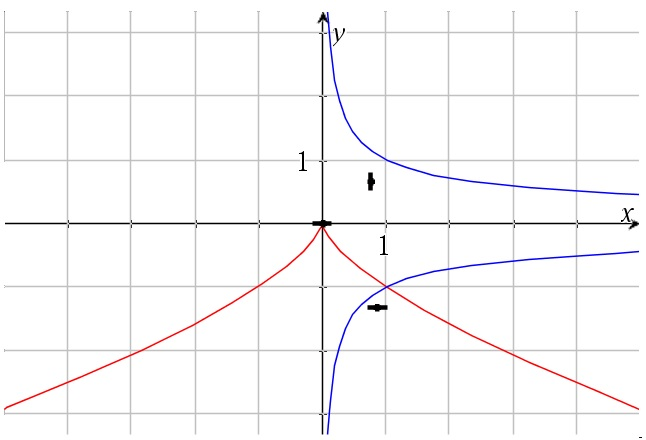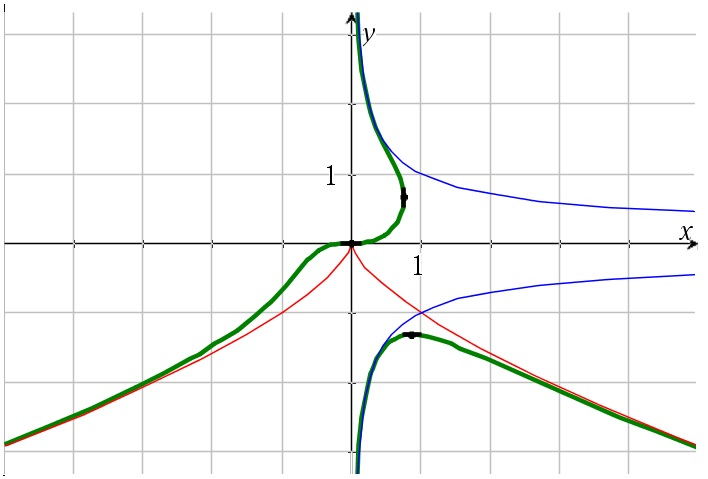# y^3+x^2=y/x

Up a level : Differentiation, derivatives
Previous page : y^3+y^2=(x^4)y+x^2Let us look at${y^3} + {x^2} = \frac{y}{x}$

Zeroes

Let us start with possible axes intercepts. If y=0 we get x2=0, so x=0. Multiplying all by x we get$x{y^3} + {x^3} = y$

So at x=0 we would get y=0, but the relation is not defined at that point, since we then would divide by 0, but we could expect the graph to exist in a neighborhood of that point.

Critical point

If we now differentiate the second equation with respect to x we get${y^3} + 3x{y^2}y' + 3{x^2} = y'$

For y´=0 we get${y^3} + 3{x^2} = 0$

Or$y = \sqrt{{ - 3{x^2}}} = - \sqrt{{3{x^2}}}$

We would thus expect to find the slope to be zero somewhere along that line.$\left\{ {\begin{array}{*{20}{c}} {x{y^3} + {x^3} = y} \\ {{y^3} + 3{x^2} = 0} \end{array}} \right.$

Using the second equation to eliminate y in the first we get$- 3{x^3} + {x^3} = - 2{x^3} = - \sqrt{{3{x^2}}}$

or$2{x^3} = \sqrt{{3{x^2}}}$

This gives us${2^3}{x^9} = 3{x^2}$

That obviously has one solution at x=0. Dividing through by x2 and 8 gives us${x^7} = \frac{3}{8}$

or$x = \sqrt{{\frac{3}{8}}} \approx 0.87$

(I had to grab my calculator there.)  Substituting that back into$y = - \sqrt{{3{x^2}}}$

gives us$y \approx - 1.31$

Making y´the subject in$y' = \frac{{{y^3} + 3{x^2}}}{{1 - 3x{y^2}}}$

This will be undefined, i.e. x´=0 when$1 - 3x{y^2} = 0$

or$x = \frac{1}{{3{y^2}}}$

We now substitute that into$x{y^3} + {x^3} = y$

to get$\frac{1}{{3{y^2}}}{y^3} + {\left( {\frac{1}{{3{y^2}}}} \right)^3} = y$

or$\frac{y}{3} + \frac{1}{{27{y^6}}} = y$

This gives us$9{y^7} + 1 = 27{y^7}$

or$y = \frac{1}{{\sqrt{{18}}}} \approx 0.66$

And , for that value of y,$x = \frac{1}{{3{y^2}}} \approx 0.76$

Asymptotes

If x goes toward infinity then${y^3} + {x^2} \to 0$

Why so? If x goes toward infinity and say y would go toward anything but infinity, then y/x would go toward 0, but at the same time would the left hand side go toward infinity, and that will not work. If we instead assume y goes toward infinity, then y3 would have to go toward infinity in about the same phase as x2, and therefore x would go way faster toward infinity than y, so y/x would go toward 0. We will thus (probably) have$y = - {x^{2/3}}$

As an asymptote, and that would be true in both the positive and the negative x direction, as is not too hard to argue for.

Also, if x goes toward 0, then we could either have that y would go to 0 to (but faster) so that both sides goes toward 0 (as we already saw is plausible from the argument about the zeroes in the beginning). Alternatively y might go toward infinity so that${y^3} + {x^2} = \frac{y}{x}$

Goes toward${y^3} = \frac{y}{x}$

or$y = \pm \frac{1}{{\sqrt x }}$

As often is, it is rather hard to find the zeroes of the second derivative, so I will not even try to find the points of intersection. Anyhow, putting these facts together we get:The solid lines are the asymptotes, and the dark lines are points with known slopes.

Before looking at the actual graph, try to figure out how it looks like.

Anyhow, here it is:

.

.

.

.

.

.

.

.

.

.

The green line is our graph.Up a level : Differentiation, derivatives
Previous page : y^3+y^2=(x^4)y+x^2Last modified: Feb 16, 2018 @ 19:24